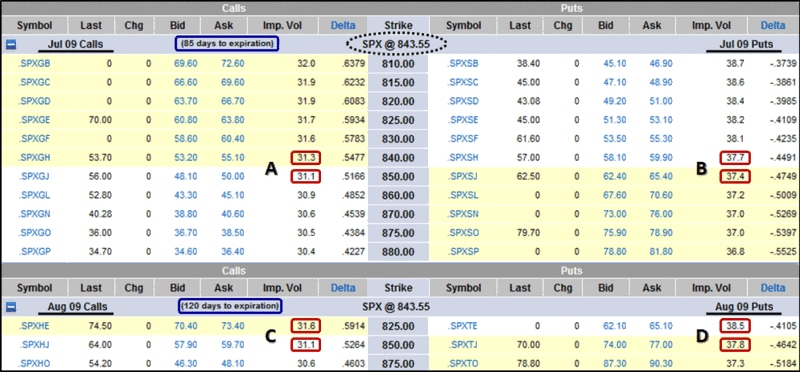## Wednesday, April 22, 2009

### How to Create Your Own Portable VXV

I recently received a question from a reader who was looking to create a homemade version of the VXV that he could apply to the Nifty (formally known as the S&P CNX Nifty), which is an index of 50 large capitalization companies on the National Stock Exchange of India. Specifically, the reader wanted to know if it would be possible to use a 93 day moving average of the India VIX to create an index that would be analogous to the VXV.

Actually, VXV ignores all historical volatility readings and utilizes implied volatility instead. It is the blending of implied volatility for the options from the months that are closest to 93 days into the future.

Refer to the graphic below and I will create a “quick and dirty” version of VXV that can be used on any underlying that has options. The example here is for the SPX, but the same process can yield a VXV proxy for any underlying.

The steps are as follows:

1. Determine the two options expiration months whose time to expiration is closest to 93 days (the term for the VXV). In this case we are using July, with 85 days until expiration and August, with 120 days until expiration. Of course once each month there will be one month with exactly 93 days until expiration.

2. Determine the last close in the SPX and the series immediately above and below the last close for each of the two months. Here the last close is 843.55. For July, the series for the calculations are 840 and 850. For August, we use 825 and 850.

3. Determine the implied volatility levels for both the puts and calls for the relevant series for each of the two months. These are highlighted in red in the optionsXpress graphic below.

4. Interpolate the implied volatility for the SPX close from the two July calls (A), July puts (B), August calls (C) and August puts (D). In this case the interpolation is done by assuming the proportions for the SPX close are the same as they are for the implied volatility values. For example:
A = 31.1 + (6.45/10)*(31.3-31.1)
A = 31.229

5. Now that we have implied volatility levels for the SPX close, we need to average these across both puts and calls for each month. July = (A + B) / 2 and August = (C + D) / 2

6. Finally, interpolate the July and August results from the step above to estimate 93 day implied volatility. The easiest way to do this is to start with the nearer month and then add the appropriate fractional portion of the later month that lifts the blended average to 93. The approach is analogous to the interpolation above and yields and equation that looks like this:
VXV = [(A + B) / 2] + [(93-85)/(120-85) * ((C + D) / 2) – ((A + B) / 2)]

This is not the same approach that is used by the CBOE, which is more complex and is detailed in the CBOE S&P 500 3-Month Volatility Index Description. This quick and dirty variant can be calculated quickly and is portable across any underlying with options, including the Nifty.[source: optionsXpress]